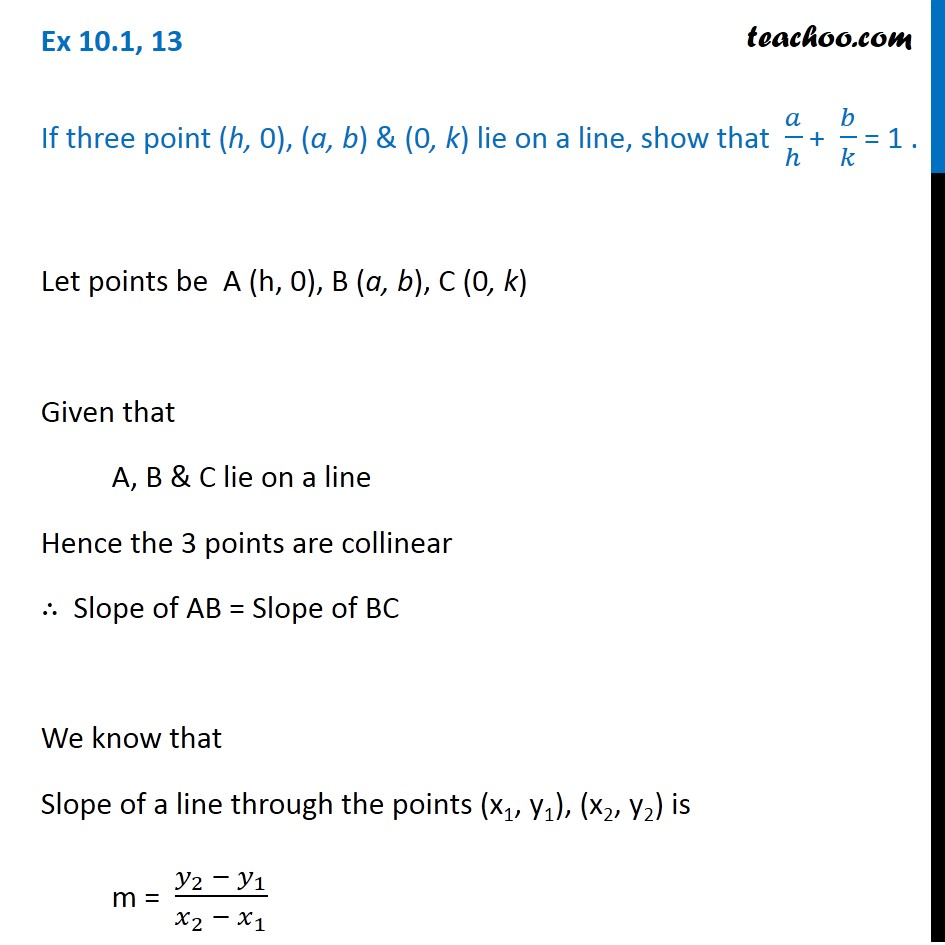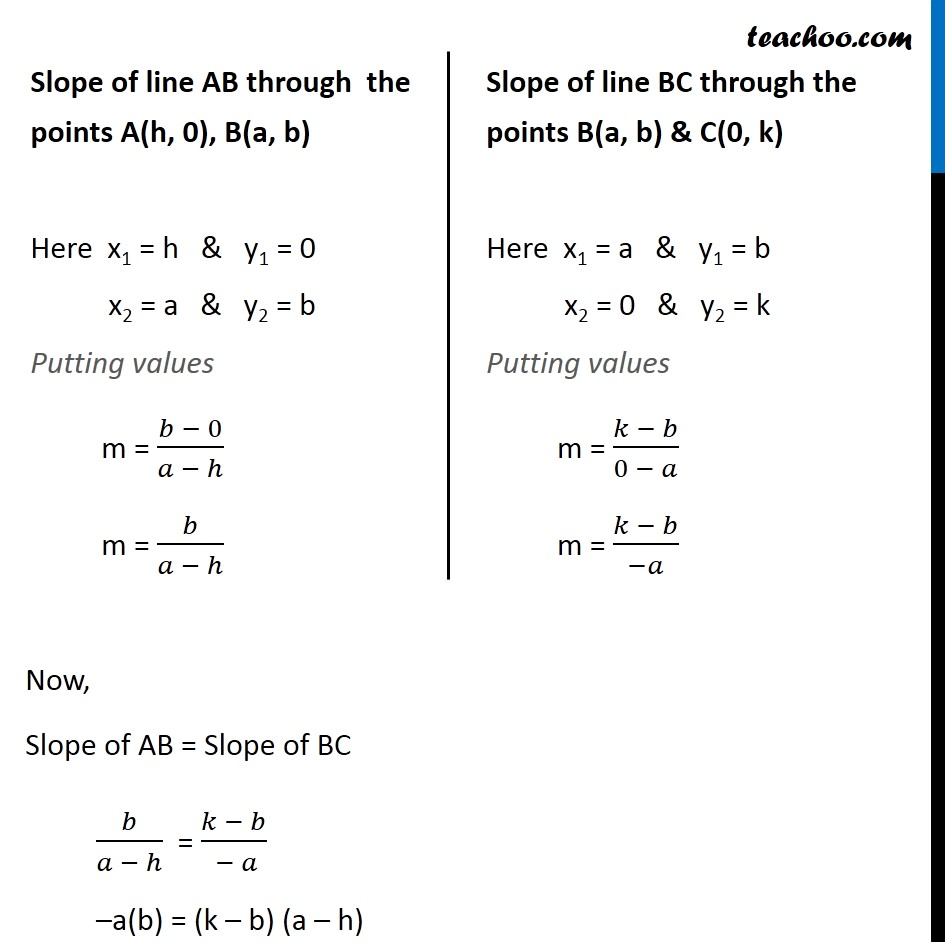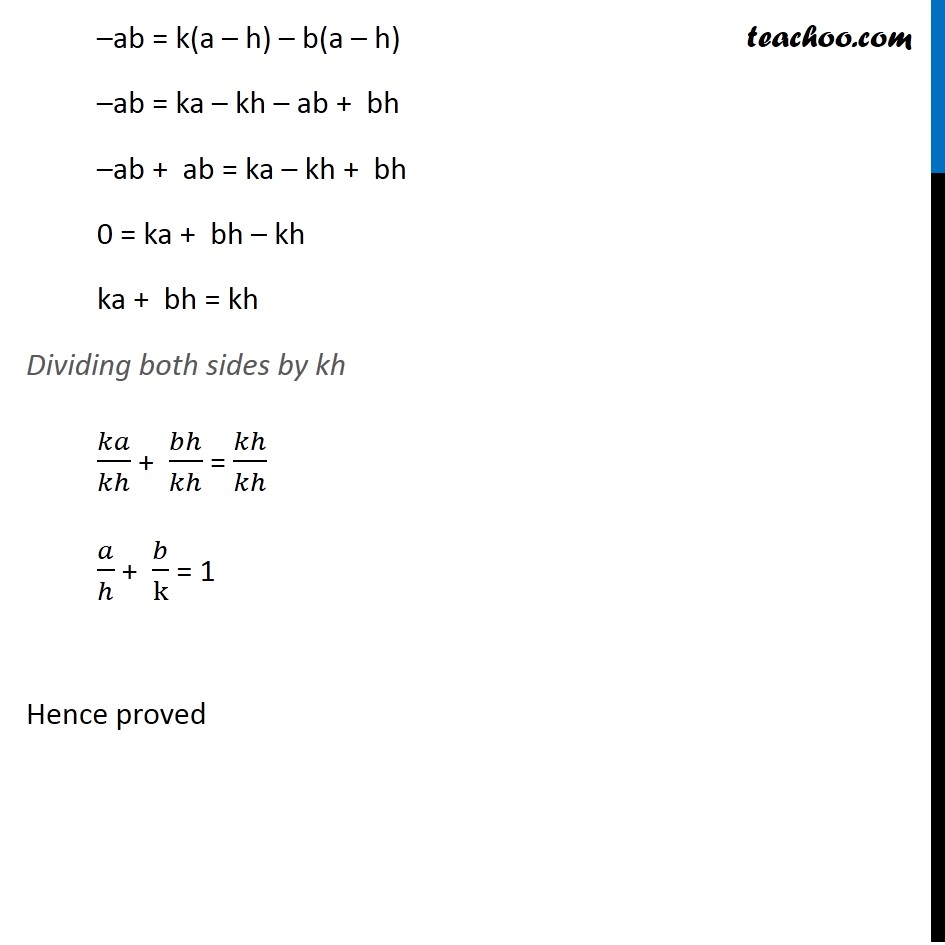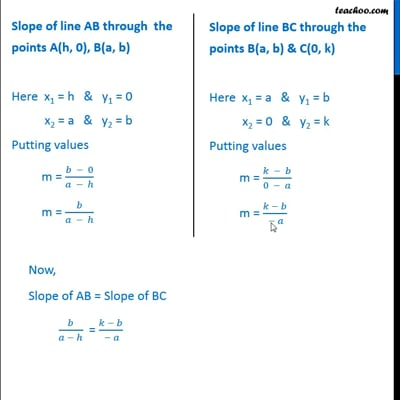Ex 10.1

Chapter 10 Class 11 Straight Lines (Term 1)
Serial order wiseThis video is only available for Teachoo black users

### Transcript

Ex 10.1, 13 If three point (h, 0), (a, b) & (0, k) lie on a line, show that 𝑎/ℎ + 𝑏/𝑘 = 1 . Let points be A (h, 0), B (a, b), C (0, k) Given that A, B & C lie on a line Hence the 3 points are collinear ∴ Slope of AB = Slope of BC We know that Slope of a line through the points (x1, y1), (x2, y2) is m = (𝑦_2 − 𝑦_1)/(𝑥_2 − 𝑥_1 ) Slope of line AB through the points A(h, 0), B(a, b) Here x1 = h & y1 = 0 x2 = a & y2 = b Putting values m = (𝑏 − 0)/(𝑎 − ℎ) m = 𝑏/(𝑎 − ℎ) Slope of line BC through the points B(a, b) & C(0, k) Here x1 = a & y1 = b x2 = 0 & y2 = k Putting values m = (𝑘 − 𝑏)/(0 − 𝑎) m = (𝑘 − 𝑏)/(−𝑎) Now, Slope of AB = Slope of BC 𝑏/(𝑎 − ℎ) = (𝑘 − 𝑏)/( − 𝑎) –a(b) = (k – b) (a – h) –ab = k(a – h) – b(a – h) –ab = ka – kh – ab + bh –ab + ab = ka – kh + bh 0 = ka + bh – kh ka + bh = kh Dividing both sides by kh 𝑘𝑎/𝑘ℎ + 𝑏ℎ/𝑘ℎ = 𝑘ℎ/𝑘ℎ 𝑎/ℎ + 𝑏/k = 1 Hence proved